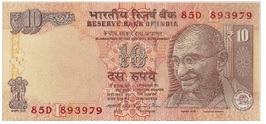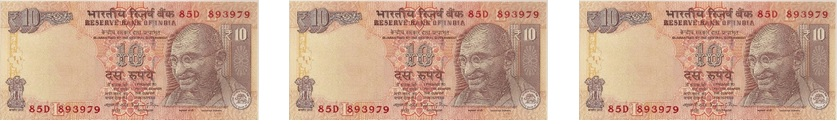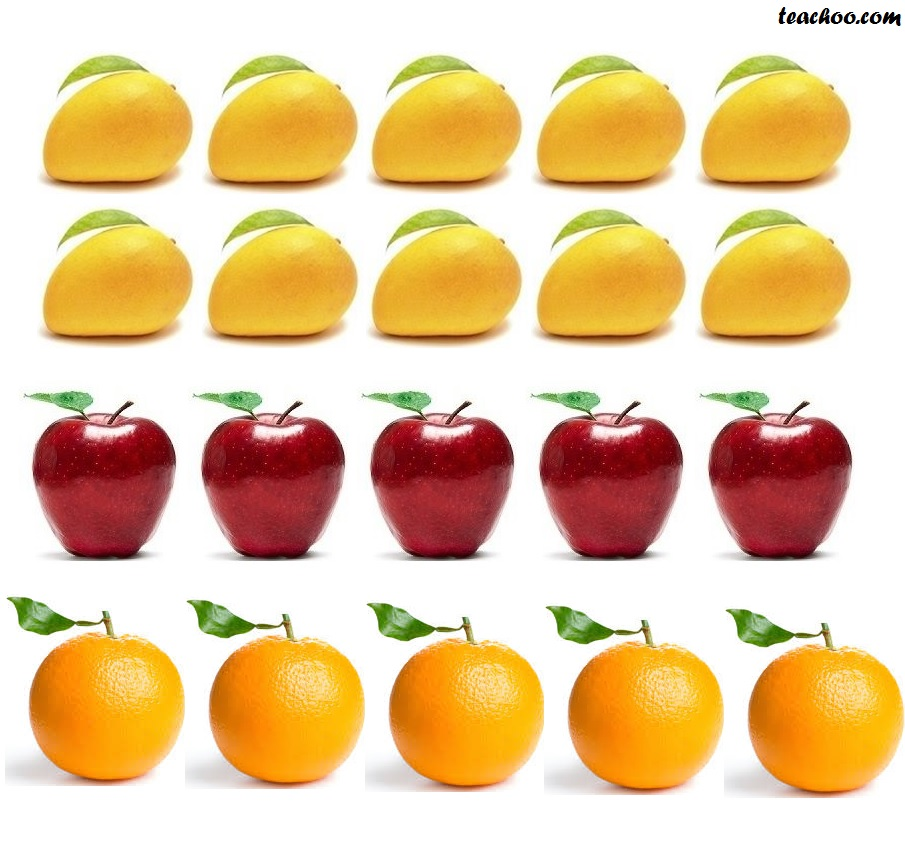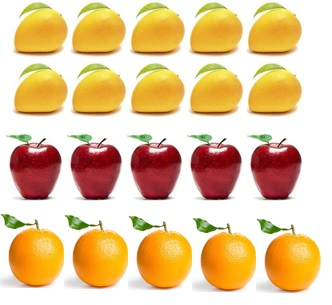Ratios

Chapter 12 Class 6 Ratio and Proportion
Concept wise

Suppose I have 10 rupees& Sanjay has 30 rupeesI can say,

Sanjay has 20 rupees more than me

And I can also say,

Sanjay has 3 times as much money than me

While writing 3 times,

I divided Sanjay’s money to my money

(Sanjay′s Money)/(My Money) = 30/10

= 3/1

Here 3/1 is ratio

We also write is as 3 : 1

Let’s take some more examples

Suppose I have 10 kg mangoes, 5 kg apples & 5 kg oranges.#### What is ratio of weight of mangoes to weight of apples:-

Dividing weight of mangoes

by weight of apple

• (Weight of mangoes)/(Weight of apple) = (10 kg)/(5 kg)
• = 10/5
• = 2/1
• Hence, 2/1 or 2 : 1 is the ratio#### Ratio of weight of apples and weight of oranges :-

Dividing weight of apples

by weight of orange(Weight of apple)/(Weight of oranges) = (5 kg)/(5 kg)

= 5/5

= 1/1

Hence, 1/1 or 1 : 1 is the ratio

#### Ratio of weight of oranges and weight of mangoes :-

Dividing weight of oranges

by weight of mangoes(Weight of oranges)/(Weight of mangoes) = (5 kg)/(10 kg)

= 5/10

= 1/2

Hence, 1/2 or 1 : 2 is the ratio

Ratio’s can work the opposite way as well

#### Suppose ratio of girls to boys in a class is 1 : 2. And, number of boys is 20. Find number of girls & total number of students.

Given ratio is 1 : 2

i.e.

(Number of girls)/(Number of boys) = 1/2

(Number of girls)/20 = 1/2

Number of girls = 20/2

Number of girls = 10

Also,

Total number of students

= Number of girls + Number of boys

= 10 + 20

= 30 Students

∴ Total number of students in the class = 30

Learn in your speed, with individual attention - Teachoo Maths 1-on-1 Class